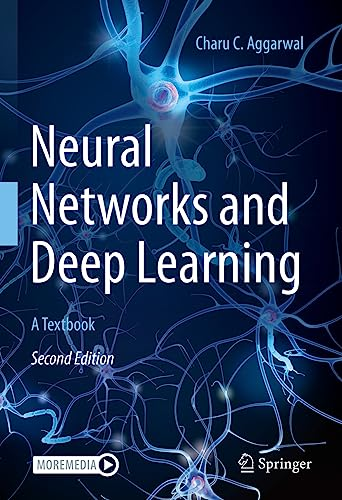# Neural Networks and Deep Learning: A Textbook, 2nd Edition

• Length: 553 pages
• Edition: 2
• Publisher:
• Publication Date: 2023-06-30
• ISBN-10: 3031296419
• ISBN-13: 9783031296413
4.3
Description

This textbook covers both classical and modern models in deep learning and includes examples and exercises throughout the chapters. Deep learning methods for various data domains, such as text, images, and graphs are presented in detail.  The chapters of this book span three categories:

The basics of neural networks: The backpropagation algorithm is discussed in Chapter 2.

Many traditional machine learning models can be understood as special cases of neural networks. Chapter 3 explores the connections between traditional machine learning and neural networks. Support vector machines, linear/logistic regression, singular value decomposition, matrix factorization, and recommender systems are shown to be special cases of neural networks.

Fundamentals of neural networks:  A detailed discussion of training and regularization is provided in Chapters 4 and 5. Chapters 6 and 7 present radial-basis function (RBF) networks and restricted Boltzmann machines.

Advanced topics in neural networks:  Chapters 8, 9, and 10 discuss recurrent neural networks, convolutional neural networks, and graph neural networks. Several advanced topics like deep reinforcement learning, attention mechanisms, transformer networks, Kohonen self-organizing maps, and generative adversarial networks are introduced in Chapters 11 and 12.

The textbook is written for graduate students and upper under graduate level students. Researchers and practitioners working within this related field will want to purchase this as well.

Where possible, an application-centric view is highlighted in order to provide an understanding of the practical uses of each class of techniques.

The second edition is substantially reorganized and expanded with separate chapters on backpropagation and graph neural networks. Many chapters have been significantly revised over the first edition.

Greater focus is placed on modern deep learning ideas such as attention mechanisms, transformers, and pre-trained language models.